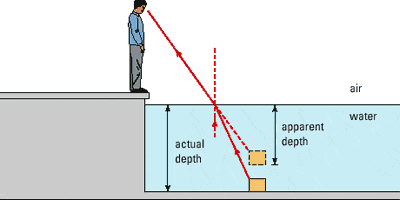# Apparent depth and snells law

## Homework Statement

A fish is 80 cm below the surface of a pond. What is the apparent depth (in cm) when viewed from a position almost directly above the fish? (For water, n = 1.33.)

## Homework Equations

Snell's law: $$n_1 sin \theta_1 = n_2 sin \theta_2$$

## The Attempt at a Solution

So far I have found the angle of refraction in the water using Snell's law. Since they are asking for "a position almost directly above the fish", I took $$\theta_1 = 90$$.

$$1= 1.33 sin \theta_2$$

$$\theta_2 = 48.75$$

But I what else can I do?? I'm really stuck! I need to find the difference between he apparent and the real depth x, 80-x=apparent length. But how? Here's a diagram which shows a similar situation:The correct answer is 60 cm.

ehild
Homework Helper
The angle in Snell's law is measured from the normal of the water surface, and it is near zero now.

ehild

The angle in Snell's law is measured from the normal of the water surface, and it is near zero now.

ehild

If this angle is zero, then what do I need to do to find the apparent depth?

ehild
Homework Helper
It is not zero, just small.

ehild

Oh, okay. How does this help us to determine the apparent depth?

ehild
Homework Helper
Look at the figure and find some right triangles.

ehild